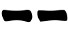# Three long dice (3d4)

Lawrence P Kaster, created these three long dice to cast I Ching hexagrams. Two of them are marked (2,2,3,3) the other one is marked (2,3,3,3). Lawrence sells them on Etsy in many variations together with other I Ching related creations.
I don't have them but from the images I feel they are nice to the touch and really pleasant to throw while casting hexagrams.

The method is rather intuitive:
1. Roll the three dices
2. Sum the face values and draw the line according the following table:
 6 7 8 93. Repeat steps 1-2 other five times drawing the hexagram from bottom to top.

#### Probabilities

Assuming the first die is the one marked (2,3,3,3)  we have the following possibilities:
6 = (2+2+2)
9 = (3+3+3)
8 = (2+3+3) or (3+3+2) or (3+2+3)
7 = (2+2+3) or (2+3+2) or (3+2+2)
that lead to the following probabilities:
Prob(6) = 1/4 * 2/4 * 2/4 = 4/64 = 1/16
Prob(9) = 3/4 * 2/4 * 2/4 = 12/64 = 3/16
Prob(8) = (1/4 * 2/4 * 2/4) + (3/4 * 2/4 * 2/4) + (3/4 * 2/4 * 2/4) = 28/64 = 7/16
Prob(7) = (1/4 * 2/4 * 2/4) + (1/4 * 2/4 * 2/4) + (3/4 * 2/4 * 2/4) = 20/64 = 5/16
Prob(yin) = Prob(yang) = 1/2
which are the same as the yarrow stalk method.

To get the three coins method probablities, the three dice should have all be marked with (2,2,3,3).